# Kinematics 1D- JEE Main Previous Year Questions with Solutions

JEE Main Previous Year Question of Physics with Solutions are available here. Practicing JEE Main Previous Year Papers Questions of Physics will help all the JEE aspirants in realizing the question pattern as well as help in analyzing their weak & strong areas.

Get detailed Class 11th &12th Physics Notes to prepare for Boards as well as competitive exams like IIT JEE, NEET etc.

eSaral helps the students in clearing and understanding each topic in a better way. eSaral is providing complete chapter-wise notes of Class 11th and 12th both for all subjects.

Besides this, eSaral also offers NCERT Solutions, Previous year questions for JEE Main and Advance, Practice questions, Test Series for JEE Main, JEE Advanced and NEET, Important questions of Physics, Chemistry, Math, and Biology and many more.

Simulator Previous Years AIEEE/JEE Mains Questions
Q. A particle has an initial velocity of $3 \hat{i}+4 \hat{j}$ and an acceleration of $0.4 \hat{\mathrm{i}}+0.3 \hat{\mathrm{j}}$. Its speed after 10s is :- (1) 7 units (2) 8.5 units (3) 10 units (4) $7 \sqrt{2}$ units [AIEEE-2009]
Ans. (4) v = u + at u = 3i + 4j + (0.4 i + 0.3 j) × 10 = 3i + 4j + 4i + 3i u = 7i + 7j $|\overrightarrow{\mathrm{u}}|=\sqrt{7^{2}+7^{2}}$ $=7 \sqrt{2}$
Q. An object, moving with a speed of 6.25 m/s, is decelerated at a rate given by $\frac{\mathrm{dv}}{\mathrm{dt}}=-2.5 \sqrt{\mathrm{v}}$ where v is the instantaneous speed. The time taken by the object, to come to rest, would be :- (1) 4 s (2) 8 s (3) 1 s (4) 2 s [AIEEE-2011]
Ans. (4) $\overrightarrow{\mathrm{a}}=\frac{\mathrm{d} \mathrm{v}}{\mathrm{dt}}$ $\frac{\mathrm{d} \mathrm{v}}{\mathrm{dt}}=-2.5 \sqrt{\mathrm{v}}$ $\int \mathrm{d} \mathrm{v} \times \mathrm{v}^{-1 / 2}=\int-2.5 \mathrm{dt}$ $\frac{\mathrm{v}^{\frac{1}{2}}}{\frac{1}{2}}=-2.5 \mathrm{t}$ $2 \sqrt{\mathrm{v}}=-2.5 \mathrm{t}+\mathrm{c}$ $2 \sqrt{6.25}=-2.5 \mathrm{t}+\mathrm{c}$ 2 × 0.25 = c 5 = c $2 \sqrt{\mathrm{v}}=-\mathrm{kt}+5$ 0 = –kt + 5 kt = 5 $\mathrm{t}=\frac{5 \mathrm{sec}}{2.5}=2 \mathrm{sec}$
Q. From a tower of height H, a particle is thrown vertically upwards with a speed u. The time taken by the particle, to hit the ground, is n times that taken by it to reach the highest point of its path. The relation between H, u and n is : (1) $2 \mathrm{g} \mathrm{H}=\mathrm{nu}^{2}(\mathrm{n}-2)$ (2) $\mathrm{g} \mathrm{H}=(\mathrm{n}-2) \mathrm{u}^{2}$ (3) $2 \mathrm{g} \mathrm{H}=\mathrm{n}^{2} \mathrm{u}^{2}$ (4) $\mathrm{g} \mathrm{H}=(\mathrm{n}-2)^{2} \mathrm{u}^{2}$ [JEE-MAIN-2014]
Ans. (1) Time to reach highest point $=\mathrm{t}=\frac{\mathrm{u}}{\mathrm{g}}$ time to reach ground = nt $\mathrm{S}=\mathrm{ut}+\frac{1}{2} \mathrm{at}^{2}$ $-\mathrm{H}=\mathrm{u}(\mathrm{nt})-\frac{1}{2} \mathrm{g}(\mathrm{nt})^{2}$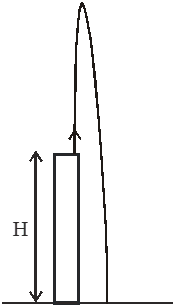$\Rightarrow 2 \mathrm{gH}=\mathrm{nu}^{2}(\mathrm{n}-2)$
Q. A body is thrown vertically upwards. Which one of the following graphs correctly represent the velocity vs time ?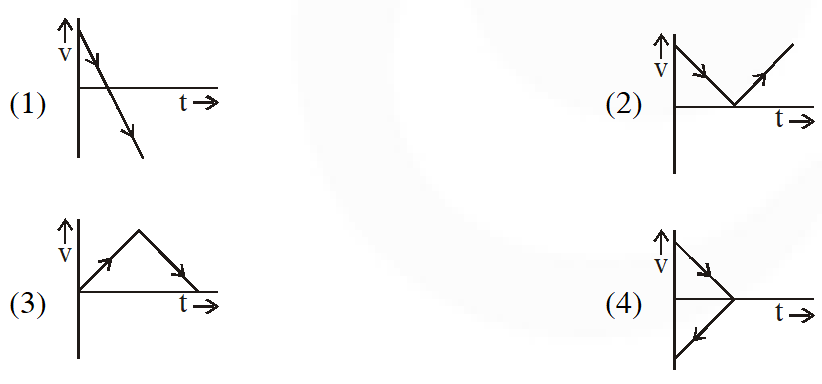[JEE Mains - 2017]
Ans. (1) Velocity at any time t is given by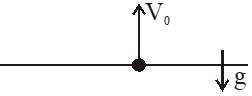v = u + at v = v0 + (–g)t $\mathrm{v}=\mathrm{v}_{0}-\mathrm{gt}$ $\Rightarrow$ straight line with negative slope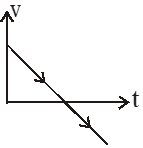Q. All the graphs below are intended to represent the same motion. One of them does it incorrectly. Pick it up.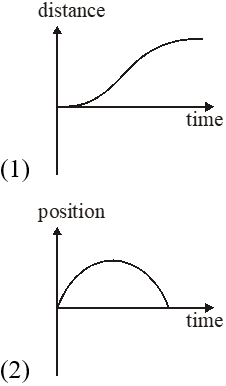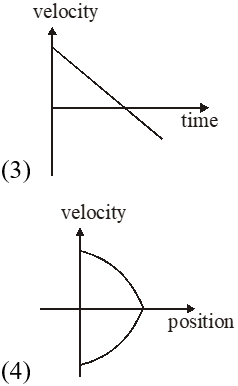[JEE Mains - 2018]
Ans. (1) In this question option (2) and (4) are the corresponding position - time graph and velocity –position graph of option (3) and its distance – time graph is given as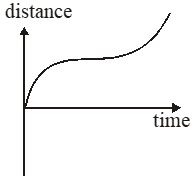Hence incorrect graph is option (1)

Rahul sahu
Sept. 24, 2021, 9:03 a.m.
potty
May 15, 2021, 11:33 p.m.
PLS ADD MORE QUESTIONS , ELSE PLS DIE You miss 100% of the shots you don't take." - ... "Whether you think you can or you think you can't, you're right." - ... "I have learned over the years that when one's mind is made up, this diminishes fear." - ... "I alone cannot change the world, but I can cast a stone across the water to create many ripples." -
mr.random
April 21, 2021, 8:54 a.m.
yes we all know u are fucking ur parents hard earned money and later ur future is going to fuck u
Pankaj
March 15, 2021, 9:32 a.m.
Nice
Anonymous
March 2, 2021, 7:24 p.m.
fuck exams
Harshit Sharma
Feb. 17, 2021, 2:20 p.m.
these are not enough for practise but uploads some more questions i really need of more privious questions
Chahat
Dec. 2, 2020, 10:03 a.m.
Yes
C.K. Harshini Lalitaa
Nov. 28, 2020, 6:09 p.m.
Very good but would have been better if updated
Pamidimukkala
Nov. 2, 2020, 10:18 p.m.
Well, even the website's name is 'e-Saral'. Way too easy.
anupama
Oct. 18, 2020, 4:17 p.m.
more and harder questions please but good
JEEBAN KUMAR BARIK.🥰
Oct. 10, 2020, 6:47 a.m.
Well/but not hard is pick up/plz update harder omes🙏🙏🙏🙏
शिवांग
Oct. 2, 2020, 8:44 a.m.
Kavita
Sept. 27, 2020, 11:06 p.m.
Anshika tiwari
Sept. 14, 2020, 8:36 a.m.
I want more previous year questions for jee mains
Abhinav anand
Sept. 1, 2020, 8:05 a.m.
Sir pls launch crash course for jee 2021
Pankaj
Sept. 1, 2020, 6:21 a.m.
😘😘
Sasi
Aug. 31, 2020, 10:56 a.m.
I want more queries
Varsha
Aug. 30, 2020, 12:07 a.m.
Ya strength of questions are less but helpfull
Varsha
Aug. 30, 2020, 12:06 a.m.
Thanks esaral these questions are helpful for us
binod
Aug. 24, 2020, 1:21 p.m.
binod
aloo
Aug. 22, 2020, 11:51 a.m.
Italy kam questions key bhai .today jadavpur dhudke lao
chin
Aug. 22, 2020, 11:49 a.m.
good questions
jay
Aug. 16, 2020, 11:30 a.m.
tthnk you but just 5 questions!!!!
prathaesh
Aug. 10, 2020, 7:23 p.m.
too less questions
ergtge
Aug. 9, 2020, 10:56 a.m.
couldn't understand last one alone
Geetika manker
Aug. 7, 2020, 9:50 a.m.
Nice but less questions
Sruthi
Aug. 2, 2020, 4:08 p.m.
Nice
Avni🙂
Aug. 2, 2020, 1:49 p.m.
Good but questions are less
Harsh
July 26, 2020, 10:26 p.m.
unknown
July 26, 2020, 2:56 p.m.
fine but very less questions
ftct
July 18, 2020, 10:31 a.m.
Xe
sana khan
July 14, 2020, 8:26 a.m.
good questions could u provide PDF of it
bhai
July 10, 2020, 8:58 a.m.
fuckingly less questions
Bharat
July 8, 2020, 6:18 p.m.
so less questions
Kirtn
July 4, 2020, 8:49 p.m.
Very less questions
July 2, 2020, 9:50 p.m.
Thank you for providing such a good questions Please can you make a pdf of it.
name
June 30, 2020, 1:09 a.m.
great effort
Rohit
June 22, 2020, 3:14 p.m.
Best
SHIVARAJ FROM KARNATAKA
June 18, 2020, 1:40 p.m.
SUPER
anonymous
June 16, 2020, 10:30 a.m.
too few questions
Anonymous
June 14, 2020, 12:43 p.m.
Anika Malhotra
May 27, 2020, 10:10 a.m.
Plz give more questions.
Paris
May 27, 2020, 8:23 a.m.
Can’t u give more questions?
May 24, 2020, 4:48 p.m.
Good job
Khushi
May 20, 2020, 8:40 p.m.
Plz give more questions..
shivom
May 1, 2020, 11:09 p.m.
Mani
April 14, 2020, 2:45 p.m.